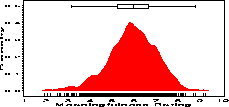density density - Nonparametric density estimates from a sample. density

# SAS Macro Programs: density

\$Version: 1.1 (28 May 2004)
Michael Friendly
York University

## DENSITY macro (get density.sas)

The DENSITY macro calculates a nonparametric density estimate of a data distribution as described in "Section 3.4". The macro produces the output data set specified by the OUT= parameter. Starting with version 1.1, the macro also produces a high-resolution plot with PROC GPLOT. The output data set contains the variables DENSITY and WINDOW in addition to the variable specified by the VAR= parameter.

A typical plotting step, using the defaults, OUT=DENSPLOT and VAR=X, would be:

```proc gplot data=densplot;
plot density * X ;
symbol1 i=join v=none;
```

### Parameters

DATA=_LAST_
Name of the input data set
OUT=DENSPLOT
Name of the output data set
VAR=X
Name if the input variable (numeric)
WINDOW=
Bandwidth (H) for kernel density estimate
XFIRST=.
Smallest X value at which density estimate is computed. If XFIRST = ., the minimum value of the VAR= variable is used.
XLAST=.
Largest X value at which density estimate is computed. If XLAST = ., the maximum value of the VAR= variable is used.
XINC=.
Step-size (increment) for computing density estimates. If XINC = ., the increment is calculated as values, XINC = (XLAST-XFIRST)/60.
GPLOT=YES
Change this to NO to suppress the plot.
symbol=
SYMBOL statement to be used to define how the points are plotted. If not specified, the program uses
```    symbol1 v=star i=join c=black;
```
HAXIS=
AXIS statement for horizontal axis. Use this to change the way the horizontal axis is scaled or labelled., e.g., HAXIS=AXIS1 together with the statement,
```axis1 order=(0 to 100 by 10) label=(f=swiss);
```
to change the default plotting range and font label.
VAXIS=
AXIS statement for vertical axis
PLOTOPT=
other plot options, eg %str(areas=1) to fill the area under the curve. (For area fill, you should define the fill pattern with a PATTERN statement.)
ANNO=
The name of an optional annotate= data set to be added to the plot.
SHOWOBS=YES
If specified, the observations are indicated with short vertical lines at the bottom of the plots.

### Example

The following example shows a density display of the distribution of rated meaningfulness for a collection of 925 English words, and illustrates the use of various graphic options. The boxplot at the top of the figure is drawn using the boxaxis macro, giving an alternative display of the distribution. The offset option on the axis1 statement allows white space at the bottom of the graph for the observation markers drawn as a result of the SHOROBS=YES option.
```axis1 order=(0 to .5 by .1) label=(a=90 'Density')
offset=(3,);
pattern1 v=solid c=red;
symbol1 v=none i=join c=black;

%boxaxis(data=paivio, out=boxanno, var=meaning, pos=96, boxwidth=5);
%density(data=paivio, var=meaning, out=densplot,
vaxis=axis1, haxis=axis2,
symbol=symbol1,
anno=boxanno,
plotopt=%str(areas=1));

```